Download Presentation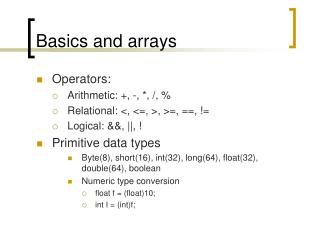Basics and arrays

# Basics and arrays - PowerPoint PPT Presentation

Basics and arrays. Operators: Arithmetic: +, -, *, /, % Relational: <, <=, >, >=, ==, != Logical: &&, ||, ! Primitive data types Byte(8), short(16), int(32), long(64), float(32), double(64), boolean Numeric type conversion float f = (float)10; int I = (int)f;. Control structure.I am the owner, or an agent authorized to act on behalf of the owner, of the copyrighted work described.
Download Presentation## Basics and arrays

An Image/Link below is provided (as is) to download presentation

Download Policy: Content on the Website is provided to you AS IS for your information and personal use and may not be sold / licensed / shared on other websites without getting consent from its author.While downloading, if for some reason you are not able to download a presentation, the publisher may have deleted the file from their server.

- - - - - - - - - - - - - - - - - - - - - - - - - - E N D - - - - - - - - - - - - - - - - - - - - - - - - - -
Presentation Transcript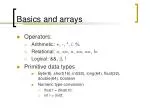Basics and arrays
• Operators:
• Arithmetic: +, -, *, /, %
• Relational: <, <=, >, >=, ==, !=
• Logical: &&, ||, !
• Primitive data types
• Byte(8), short(16), int(32), long(64), float(32), double(64), boolean
• Numeric type conversion
• float f = (float)10;
• int I = (int)f;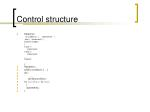Control structure
• Selection

if( condition ) { statements; }

else { statements; }

Switch( integer)

{

Case 1:

statements;

Case 2:

statements;

Default:

..

}

• Repetition
• while( condition) { … }
• do {
• ….
• }while(condition);
• for ( x = 0; x < 10; x++)
• {
• statements;
• }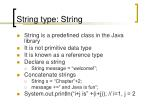String type: String
• String is a predefined class in the Java library
• It is not primitive data type
• It is known as a reference type
• Declare a string
• String message = “welcome!”;
• Concatenate strings
• String s = “Chapter”+2;
• message +=“ and Java is fun”;
• System.out.println(“i+j is” +(i+j)); // i=1, j = 2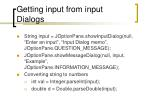Getting input from input Dialogs
• String input = JOptionPane.showInputDialog(null, “Enter an input”, “Input Dialog memo”, JOptionPane.QUESTION_MESSAGE);
• JOptionPane.showMessageDialog(null, input, “Example”, JOptionPane.INFORMATION_MESSAGE);
• Converting string to numbers
• int val = Integer.parseInt(input);
• double d = Double.parseDouble(input);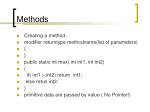Methods
• Creating a method:
• modifier returntype methodname(list of parameters)
• {
• }
• public static int max( int int1, int int2)
• {
• if( int1 > int2) return int1;
• else retun int2;
• }
• primitive data are passed by value ( No Pointer!)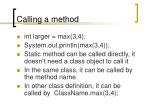Calling a method
• int larger = max(3,4);
• System.out.println(max(3,4));
• Static method can be called directly, it doesn’t need a class object to call it
• In the same class, it can be called by the method name
• In other class definition, it can be called by ClassName.max(3,4);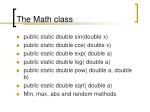The Math class
• public static double sin(double x)
• public static double cos( double x)
• public static double exp( double a)
• public static double log( double a)
• public static double pow( double a, double b)
• public static double sqrt( double a)
• Min, max, abs and random methods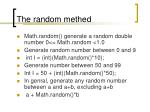The random methed
• Math.random() generate a random double number 0<= Math.random <1.0
• Generate random number between 0 and 9
• int I = (int)(Math.random()*10);
• Generate number between 50 and 99
• Int I = 50 + (int)(Math.random()*50);
• In genral, generate any random number between a and a+b, excluding a+b
• a + Math.random()*b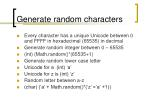Generate random characters
• Every character has a unique Unicode between 0 and FFFF in hexadecimal (65535) in decimal
• Generate random integer between 0 – 65535
• (int) (Math.random()*(65535+1)
• Generate random lower case letter
• Unicode for a (int) ‘a’
• Unicode for z is (int) ‘z’
• Random letter between a-z
• (char) (‘a’ + Math.random()*(‘z’ =‘a’ +1))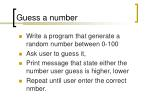Guess a number
• Write a program that generate a random number between 0-100
• Ask user to guess it,
• Print message that state either the number user guess is higher, lower
• Repeat until user enter the correct nmber.